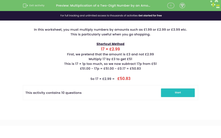# Multiply Amounts of Money by a Two-Digit Number

In this worksheet, students will use a shortcut method to multiply amounts such as £3.99 by a two-digit number.Key stage:  KS 3

Curriculum topic:   Number

Curriculum subtopic:   Use Standard Units of Measure

Difficulty level:#### Worksheet Overview

In this activity, we will multiply numbers by amounts such as £1.99 or £2.99 or £3.99 etc.

This is particularly useful when you go shopping.

Shortcut Method

17 × £2.99

First, we pretend that the amount is £3 and not £2.99

Multiply 17 by £3 to get £51

This is 17 × 1p too much, so we now subtract 17p from £51

£51.00 - 17p = £51.00 - £0.17 = £50.83

So 17 × £2.99 =   £50.83Alternatively, we can just do our normal multiplication calculation by removing the decimal point, doing the multiplication and then putting the decimal point back in, making sure that we have the same number of decimal places in our answer as in the original calculation.

17 x £2.99 had two decimal places.

17 x 299 = 5083

Put the decimal point back in to give two decimal places: 50.83

Note that answers will have two decimal places if we are doing calculations with money!

Let's have a go at these questions now.

### What is EdPlace?

We're your National Curriculum aligned online education content provider helping each child succeed in English, maths and science from year 1 to GCSE. With an EdPlace account you’ll be able to track and measure progress, helping each child achieve their best. We build confidence and attainment by personalising each child’s learning at a level that suits them.

Get started